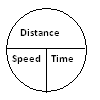# Solution

My friend and I are facing opposite directions when we begin to walk. It is a circular track. So, it implies we are walking towards each other, meet at a point, and, then, continue the cycle. Since we are walking towards each other, our speeds get added. For example, if my speed is 2 kilometres per hour and my friend's speed is 3 kilometres per hour, then in one hour we, together, cover a distance of 5 kilometres while walking towards each other. Suppose the total distance is 10 kilometres, then in one hour we, together, walk 5 kilometres and we are now separated by 5 kilometres.

That is the case here too. Our speeds are in the ratio 2:3, so our collective relative speed is 2+3 = 5 rounds per hour. So in one hour, we together make five rounds of the circular track and, therefore, cross each other five times. In 30 minutes, we make two complete rounds and so cross each other twice. Therefore, in one-and-half hours we cross each other seven times.Now, if I really want to know when we cross each other, I would prefer to draw a pie chart. I find this convenient. It helps me to keep track of things, while keeping in mind that distance = speed x time. So everytime I need to find the time, I draw a pie chart and after substituting the values for distance and speed, I find the time.

Since the speeds have been given as a ratio and no actual distance has been mentioned, I think I can conveniently assume a distance. It doesn't matter because the speeds will work out accordingly.

Let me assume that the circumference of the circular track is 600 metres. Then my speed is 1200 metres per hour (I make two rounds per hour) and my friend's speed is 1800 metres (he makes three rounds per hour).

So our collective speed is 1200 + 1800 = 3000 metres per hour. We will first meet when, together, we have covered 600 metres. Therefore, distance = 600 and speed = 3000 metres per hour. Now distance = speed x time, so 600 = 3000 x t1. Thus, t1 = 1/5 hours, or 12 minutes. So after we start walking, we will meet for the first time after 12 minutes. Having crossed each other, we will meet again after covering another 600 metres. So for the second meeting, the total distance covered is 1200 metres. Distance = speed x time, so 1200 = 3000 x t2. Therefore t2 = 2/5 hours, or 24 minutes.

The third meeting will occur after covering 1800 metres, after 36 minutes. The fourth will occur on covering 2400 metres, after 48 minutes. This is how it goes.

Thus, if we keep walking, we will meet after 12, 24, 36, 48, 60, 72, 84, 96, 108, ...... minutes. But we have walked for one-and-half hours, that is 90 minutes. So, during this 90 minutes we have crossed each other for the last time at the 84th minute. We have crossed each other seven times - at the 12th minute, 24th minute, 36th minute, 48th minute, 60th minute, 72nd minute and 84th minute.

• Union Public Service Commission - www.upsc.gov.in
• IIT-Kharagpur - www.iitkgp.ac.in
• Indian Statistical Institute - www.isical.ac.in
• Indian Institute of Technology Madras - www.iitm.ac.in
• Indian Institute of Management, Ahmedabad - www.iimahd.ernet.in
• Indian Institute of Mass Commission - www.iimc.nic.in
• IIT Bombay - www.iitb.ac.in